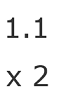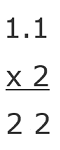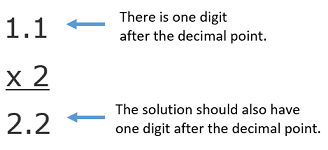# 1.1 x 2: How-to & Steps

Instructor: Mia Primas

Mia has taught math and science and has a Master's Degree in Secondary Teaching.

In this lesson, you will learn the steps for multiplying a decimal by a whole number. You will then see two methods that can be used to solve 1.1 x 2.

## Steps for Using the Column Method

Step 1: Align the numbers vertically.

Step 2: Multiply each digit of the decimal by the whole number.

Step 3: Place the decimal point.

## How to Multiply 1.1 x 2

• Step 1: Align the numbers vertically.• Step 2: Multiply each digit of the decimal by the whole number.• Step 3: Place the decimal point.

To determine where the decimal point should go, count the number of digits after the decimal point of the number being multiplied. The solution should have the same number of digits after its decimal point.## The Solution

Therefore, the solution to 1.1 x 2 is 2.2.

## Alternate Method

Instead of using the column method, an alternate method can be used that involves expanding the decimal and applying the distributive property.

• Step 1: Write the decimal in expanded form, with each digit representing its place value.

To unlock this lesson you must be a Study.com Member.

### Register to view this lesson

Are you a student or a teacher?